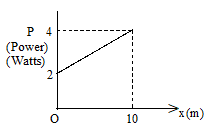Power
Question

# A particle A of mass $\frac{10}{7}$ kg is moving in the positive direction of X.  Its initial position is x=0 and initial velocity is 1m/sec.  The velocity at x = 10m is …..Moderate
Solution

## Area of the graph = $\text{∫pdx=area}$ of the trapezium =  $\frac{1}{2}\left(4+2\right)×10=30$$\text{∫pdx=30}$$\text{∫Fvdx=30}$$\text{∫}\frac{mdv}{dt}vdx=30$$\text{∫mdv.v}\frac{\mathrm{dx}}{\mathrm{dt}}=30$${\text{∫mv}}^{2}\mathrm{dv}=30$$m{\left|\frac{{v}^{3}}{3}\right|}_{1}^{u}=30$$\text{u=4m/s}$

Get Instant Solutions